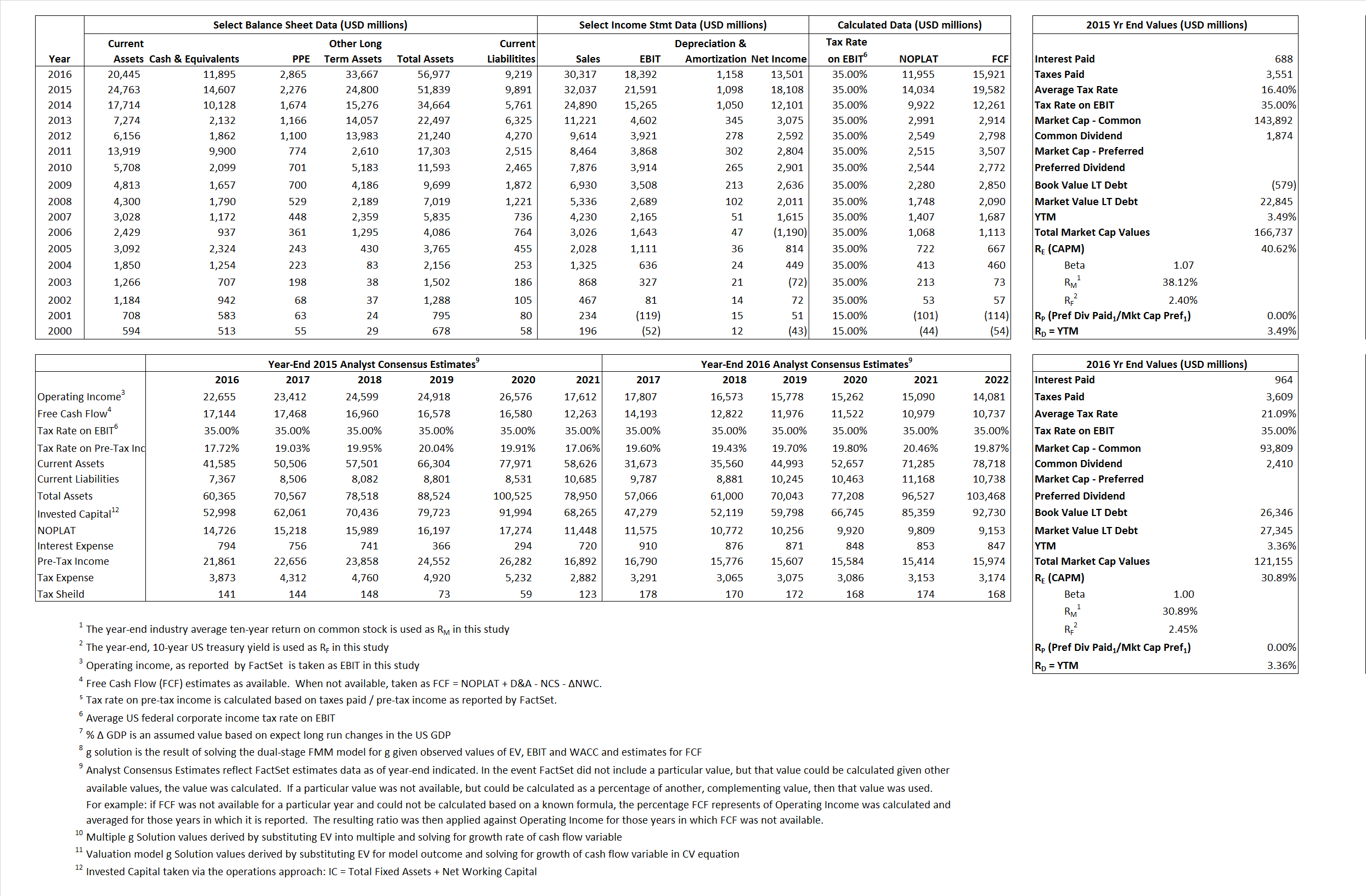## Analyst Listing

The following analysts provide coverage for the subject firm as of May 2016:

 Broker Analyst Analyst Email Jefferies Brian Abrahams babrahams@jefferies.com Piper Jaffray Joshua Schimmer joshua.e.schimmer@pjc.com RBC Capital Markets Michael J. Yee michael.yee@rbccm.com Leerink Partners Geoffrey C. Porges geoffrey.porges@leerink.com Maxim Group Jason Kolbert jkolbert@maximgrp.com Atlantic Equities Steve Chesney s.chesney@atlantic-equities.com Cowen & Company Phil Nadeau phil.nadeau@cowen.com Wells Fargo Securities Jim Birchenough jim.birchenough@wellsfargo.com Needham Alan Carr acarr@needhamco.com William Blair John Sonnier jsonnier@williamblair.com BMO Capital Markets M. Ian Somaiya ian.somaiya@bmo.com Credit Suisse Alethia Young alethia.young@credit-suisse.com Evercore ISI Mark Schoenebaum mark.schoenebaum@evercoreisi.com Guggenheim Securities Tony Butler tony.butler@guggenheimpartners.com

## Primary Input Data## Derived Input Data

### Equational Form

Net Operating Profit Less Adjusted Taxes NOPLAT  14,034  11,955$NOPLAT\, =\, EBIT\, x\, (1 \,-\, Avg \,\,Tax\,\, Rate\,\, on\,\, EBIT)$
Free Cash Flow FCF 19,582 15,921$FCF\,=NOPLAT\,+\,Non-Cash\,Expenses-\Delta NWC\,-\,NCS$
Tax Shield TS 113 203$TS\,=\,Interest\,\,Paid\,\,x\,\, Avg \,\,Tax\,\,Rate\,\, on\,\, Pre-Tax\,\, Income$
Invested Capital IC 41,948 47,758$IC\,=\,Fixed\,\,Operating\,\,Assets\,\,+\,\,Net\,\, Working\,\, Capital$
Return on Invested Capital ROIC 33.46%  25.03%$ROIC\,=\,\frac { NOPLAT }{ IC }$
Net Investment NetInv 14,143 6,968$NetInv\,=\,{ {IC}_{1}}-{{IC}_{0}}+Depreciation$
Investment Rate IR  100.78%  58.29%$IR\,=\,\frac {NetInv}{NOPLAT}$
Weighted Average Cost of Capital
WACCMarket  35.45%  24.52%$WACC\,=\,\frac { E }{ V } { R }_{ E }\,+\,\frac { P }{ V } { R }_{ P }\,+\,\frac { D }{ V } { R }_{ D }\left( 1- Avg\,\, Tax\,\,Rate\,\,on\,\,Pre-Tax\,\,Income \right)$
WACCBook   8.93%   7.54%
Enterprise value
EVMarket  152,130  109,260$EV\,=\,Market\,\,Cap\,\,Equity\,+\,\,Long\,\,Term\,\,Debt\,-\,Cash$
EVBook  128,706  108,260
Long-Run Growth
g = IR x ROIC
33.72%  14.59% Long-run growth rates of the income variable are used in the Continuing Value portion of the valuation models.
g = %$\Delta$ GDP    2.50%    2.50%
Margin from Operations M  67.39%  60.67%$M\,\,=\,\,\frac{EBIT}{SALES}$
Depreciation/Amortization Rate D  4.84%  5.92%$D\,\,=\,\,\frac{D+A}{EBITDA}$

## Valuation Multiple Outcomes

The outcomes presented in this study are the result of original input data, derived data, and synthesized inputs.

### model g solution

12/31/2015 12/31/2016 12/31/2015 12/31/2016 12/31/2015 12/31/2016

EV/SALES$\frac {EV}{Sales} \,= \,\frac{ROIC\, -\, g}{ROIC\,(WACC\,-\,g)}\,(1\,-\,T)\,(M)$

4.75  3.60  36.22%  24.12%  36.63% 24.12%

EV/EBITDA$\frac {EV}{EBITDA} \,= \,\frac{ROIC\, -\, g}{ROIC\,(WACC\,-\,g)}\,(1\,-\,T)\,(1\,-\,D)$

6.71 5.59 36.22% 24.12% 36.63% 24.12%

EV/NOPLAT$\frac {EV}{NOPLAT} \,= \,\frac{ROIC\, -\, g}{ROIC\,(WACC\,-\,g)}$

10.84 9.14 36.22% 24.12% 36.63% 24.12%

EV/FCFOPS$\frac {EV}{FCF_{OPS}} \,= \,\frac{ROIC\, -\, g}{ROIC\,(WACC\,-\,g)}\,(1\,-\,T)$

7.77 6.86 36.22% 24.12% 36.63% 24.12%

EV/EBIT$\frac {EV}{EBIT} \,= \,\frac{ROIC\, -\, g}{ROIC\,(WACC\,-\,g)}\,(1\,-\,T)$

7.05 5.94 36.22% 24.12% 36.63% 24.12%

EV/IC$\frac {EV}{IC} \,= \,\frac{ROIC\, -\, g}{WACC\,-\,g}$

3.63 2.29 36.22% 24.12% 36.63% 24.12%